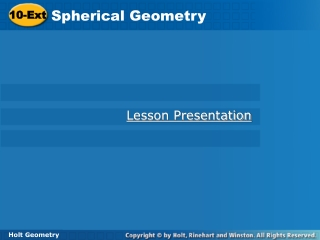# 10-Ext - PowerPoint PPT PresentationDownload Presentation10-Ext

10-ExtDownload Presentation## 10-Ext

- - - - - - - - - - - - - - - - - - - - - - - - - - - E N D - - - - - - - - - - - - - - - - - - - - - - - - - - -
##### Presentation Transcript

1. Spherical Geometry 10-Ext Lesson Presentation Holt Geometry

2. Objective Understand spherical geometry as an example of non-Euclidean geometry.

3. Vocabulary non-Euclidean geometry spherical geometry

4. Euclidean geometry is based on figures in a plane. Non-Euclidean geometryis based on figures in a curved surface. In a non-Euclidean geometry system, the Parallel Postulate is not true. One type of non-Euclidean geometry is spherical geometry, which is the study of figures on the surface of a sphere.

5. A line in Euclidean geometry is the shortest path between two points. On a sphere, the shortest path between two points is along a great circle, so “lines” in spherical geometry are defined as great circles. In spherical geometry, there are no parallel lines. Any two lines intersect at two points.

6. Caution! The two points used to name a line cannot be exactly opposite each other on the sphere. In Example 1, AB could refer to more than one line.

7. PQ is a line PQ is a segment. Example 1: Classifying Figures in Spherical Geometry Name a line, a segment, and a triangle on the sphere. ∆PQR is a triangle.

8. AD is a line AD is a segment. Check It Out! Example 1 Name a line, segment, and triangle on the sphere. ∆BCD is a triangle.

9. Imagine cutting an orange in half and then cutting each half in quarters using two perpendicular cuts. Each of the resulting triangles has three right angles.

10. Example 2A: Classifying Spherical Triangles Classify the spherical triangle by its angle measures and by its side lengths. ∆XYZ acute isosceles triangle

11. Example 2B: Classifying Spherical Triangles Classify the spherical triangle by its angle measures and by its side lengths. ∆STU on Earth has vertex S at the South Pole and vertices T and U on the equator. TU is equal to one-fourth the circumference of Earth. right equilateral triangle

12. Check It Out! Example 2 Classify ∆VWX by its angle measures and by its side lengths. ∆VWX is equiangular and equilateral.

13. The area of a spherical triangle is part of the surface area of the sphere. For the piece of orange on page 726, the area is of the surface area of the orange, or . If you know the radius of a sphere and the measure of each angle, you can find the area of the triangle.

14. Example 3A: Finding the Area of Spherical Triangles Find the area of each spherical triangle. Round to the nearest tenth, if necessary. A ≈ 62.8 cm2

15. Example 3B: Finding the Area of Spherical Triangles Find the area of the spherical triangle. Round to the nearest tenth, if necessary. ∆QRS on Earth with mQ = 78°, mR = 92°, and mS = 45°. A ≈ 9,574,506.9 mi2

16. Check It Out! Example 3 Find the area of ∆KLM on a sphere with diameter 20 ft, where mK = 90°, mL = 90°, and mM = 30°. Round to the nearest tenth. A ≈ 52.4 ft2